# How to compare data in two Google sheets or columns

Whether there's summer knocking on our doors or winter invading Westeros, we still work in Google Sheets and have to compare different pieces of tables with one another. In this article, I'm sharing ways of matching your data and giving away tips on doing that swiftly.

## Compare two columns or sheets

One of the tasks you may have is to scan two columns or sheets for matches or differences and identify them somewhere outside the tables.

### Compare two columns in Google Sheets for matches and differences

I'll start with comparing two cells in Google Sheets. This way lets you scan entire columns row by row.

#### Example 1. Google Sheets – compare two cells

For this first example, you will need a helper column in order to enter the formula into the first row of the data to compare:

`=A2=B2`

If cells match, you'll see TRUE, otherwise FALSE. To check all cells in a column, copy the formula down to other rows: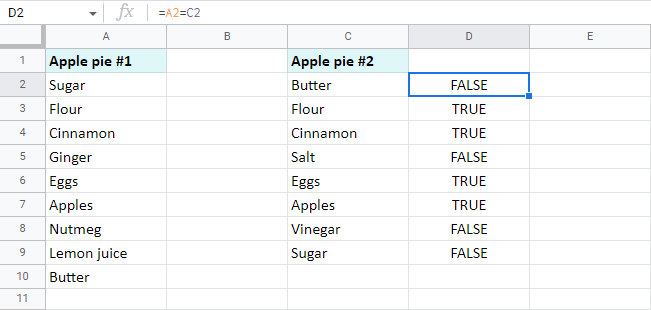Tip. To compare columns from different files, you need to use the IMPORTRANGE function:

`=A2=IMPORTRANGE("spreadsheet_url","Sheet1!A2")`

#### Example 2. Google Sheets – compare two lists for matches and differences

• A neater solution would be to use the IF function. You'll be able to set the exact status for identical and different cells:

`=IF(A2=B2,"Match","Differ")`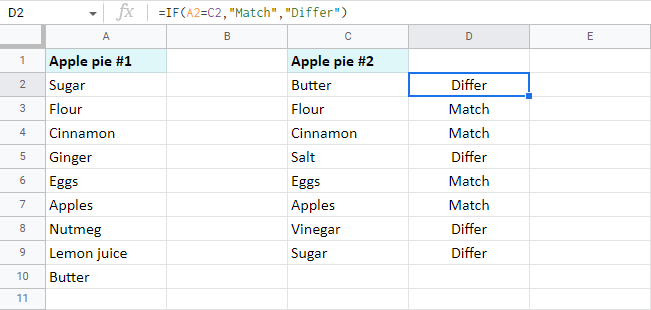Tip. If your data is written in different cases and you'd like to consider such words as different, here's the formula for you:

`=IF(EXACT(A2,B2),"Match","Differ")`

Where EXACT considers the case and looks for the complete identicals.

• To identify only rows with duplicate cells, use this formula:

`=IF(A2=B2,"Match","")`

• To mark only rows with unique records between cells in two columns, take this one:

`=IF(A2=B2,"","Differ")`

#### Example 3. Compare two columns in Google Sheets

• There's a way to avoid copying the formula over each row. You can forge an array IF formula in the first cell of your helper column:

`=ArrayFormula(IF(A2:A=C2:C,"","Differ"))`

This IF pairs each cell of column A with the same row in column C. If records are different, the row will be identified accordingly. What is nice about this array formula is that it automatically marks each and every row at once: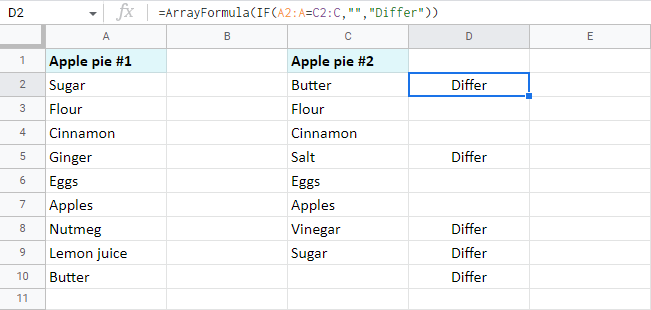• In case you'd rather name the rows with identical cells, fill the second argument of the formula instead of the third one:

`=ArrayFormula(IF(A2:A=C2:C,"Match",""))`

#### Example 4. Compare two Google Sheets for differences

Oftentimes you need to compare two columns in Google Sheets that belong inside a huge table. Or they can be entirely different sheets like reports, price lists, working shifts per month, etc. Then, I believe, you can't afford to create a helper column or it can be quite difficult to manage.

If this sounds familiar, don't worry, you can still mark the differences on another sheet.

Here are two tables with products and their prices. I want to locate all cells with different contents between these tables: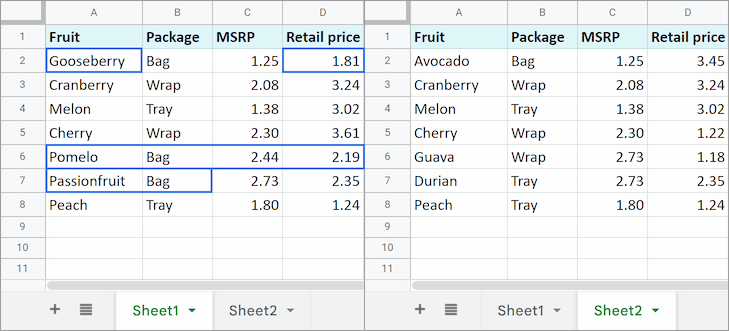Start with creating a new sheet and enter the next formula into A1:

`=IF(Sheet1!A1<>Sheet2!A1,Sheet1!A1&" | "&Sheet2!A1,"")`

Note. You must copy the formula over the range equal to the size of the biggest table.

As a result, you will see only those cells that differ in contents. The formula will also pull records from both tables and separate them with a character you enter to the formula: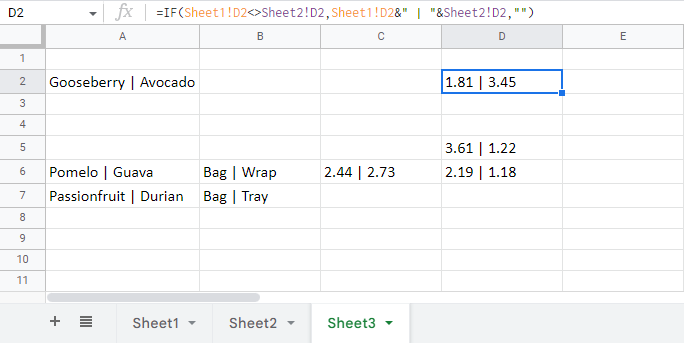Tip. If the sheets to compare are in different files, again, just incorporate the IMPORTRANGE function:

`=IF(Sheet1!A1<>IMPORTRANGE("2nd_spreadsheet_url","Sheet1!A1"),Sheet1!A1&" | "&IMPORTRANGE("2nd_spreadsheet_url","Sheet1!A1"),"")`

### Tool for Google Sheets to compare two columns and sheets

Of course, each of the examples above can be used to compare two columns from one or two tables or even match sheets themselves. However, there is a tool we created for this task that will benefit you a lot.

It will compare two Google sheets and columns for duplicates or uniques in 3 steps. Make it mark the found records with a status column (that can be filtered, by the way) or colour, copy or move them to another location, or even clear cells and delete entire rows with dupes whatsoever.

I used the add-on to find the rows from Sheet1 that are absent from Sheet2 based on Fruit and MSRP columns: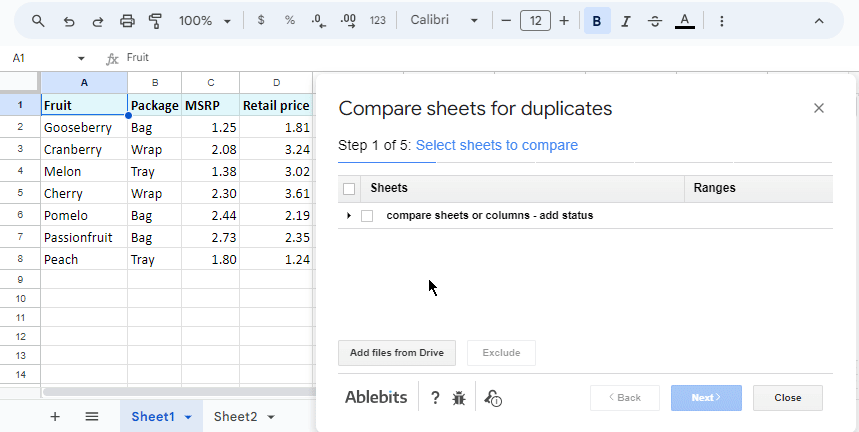For your better convenience, we've described all the tool's options on its help page and in this video:

Feel free to try it for yourself and notice how much time it saves you. :)

## Compare data in two Google Sheets and fetch missing records

Comparing two Google Sheets for differences and repeats is half the work, but what about missing data? There are special functions for this as well, for example, VLOOKUP. Let's see what you can do.

### Find missing data

#### Example 1

Imagine you have two lists of products (columns A and B in my case, but they can simply be on different sheets). You need to find those presented in the first list but not in the second one. This formula will do the trick:

`=ISERROR(VLOOKUP(A2,\$B:\$B,1,0))`

How does the formula work:

• VLOOKUP searches for the product from A2 in the second list. If it's there, the function returns the product name. Or else you will get an #N/A error meaning the value wasn't found in column B.
• ISERROR checks what VLOOKUP returns and shows you TRUE if it's the value and FALSE if it's the error.

Thus, cells with FALSE is what you're looking for. Copy the formula to other cells to check each product from the first list: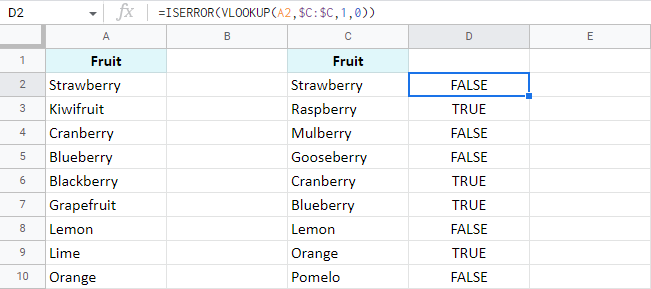Note. If your columns are in different sheets, your formula will reference one of them:

`=ISERROR(VLOOKUP(A2,Sheet2!\$B:\$B,1,0))`

Tip. To get by with a one-cell formula, it should be an array one. Such formula will automatically fill all cells with results:

`=ArrayFormula(ISERROR(VLOOKUP(A2:A10,\$B:\$B,1,0)))`

#### Example 2

Another smart way would be to count all appearances of the product from A2 in column B:

`=IF(COUNTIF(\$B:\$B, \$A2)=0, "Not found", "")`

If there's absolutely nothing to count, the IF function will mark cells with Not found. Other cells will remain empty: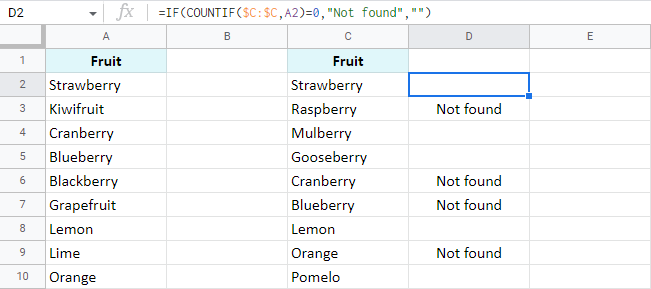#### Example 3

Where there's VLOOKUP, there's MATCH. You know that, right? ;) Here's the formula to match products rather than count:

`=IF(ISERROR(MATCH(\$A2,\$B:\$B,0)),"Not found","")`

Tip. Feel free to specify the exact range of the second column if it remains the same:

`=IF(ISERROR(MATCH(\$A2,\$B2:\$B28,0)),"Not found","")`

### Pull matching data

#### Example 1

Your task may be a bit fancier: you may need to pull all missing information for the records common for both tables, for example, update prices. If so, you'll need to wrap MATCH in INDEX:

`=INDEX(\$E:\$E,MATCH(\$A2,\$D:\$D,0))`

The formula compares fruits in column A with fruits in column D. For everything found, it pulls the prices from column E to column B.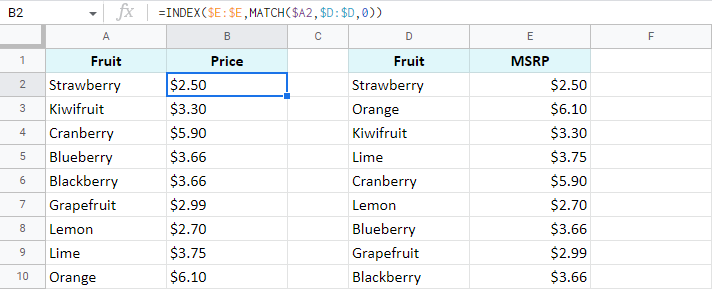#### Example 2

As you may have guessed, another example would use the Google Sheets VLOOKUP function that we described some time ago.

#### Merge sheets using the add-on

If you're tired of formulas, you can use our Merge Sheets add-on to quickly match and merge two Google sheets. Alongside its basic purpose to pull the missing data, it can also update existing values and even add non-matching rows. You can see all changes in colour or in a status column that can be filtered.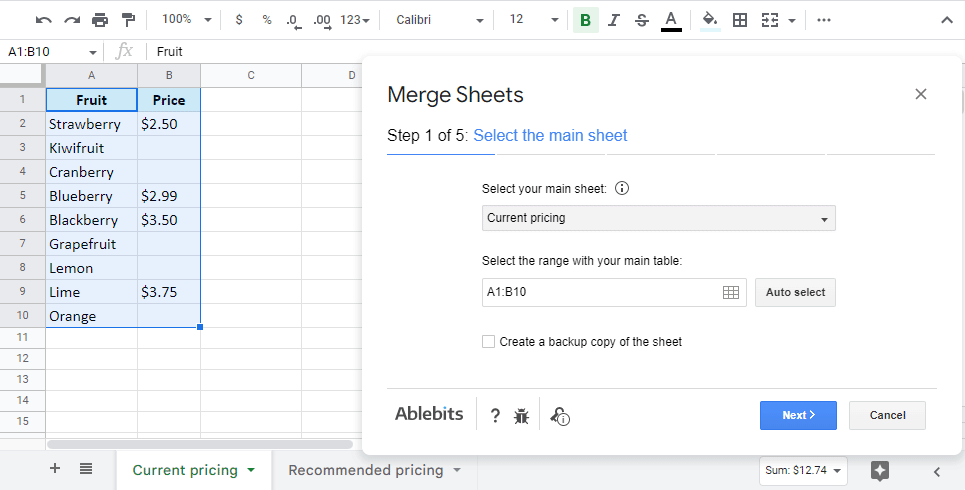Tip. Also, make sure to check out this video about the Merge Sheets add-on:

## Conditional formatting to compare data in two Google Sheets

There's one more standard way Google offers to compare your data – by colouring matches and/or differences via conditional formatting. This method makes all records you're looking for stand out instantly. Your job here is to create a rule with a formula and apply it to a correct data range.

### Highlight duplicates in two sheets or columns

Let's compare two columns in Google Sheets for matches and colour only those cells in column A that tally with cells in the same row in column C:

1. Select the range with records to color (A2:A10 for me).
3. Enter a simple formula to the rule:

`=A2=C2`

4. Pick the color to highlight cells.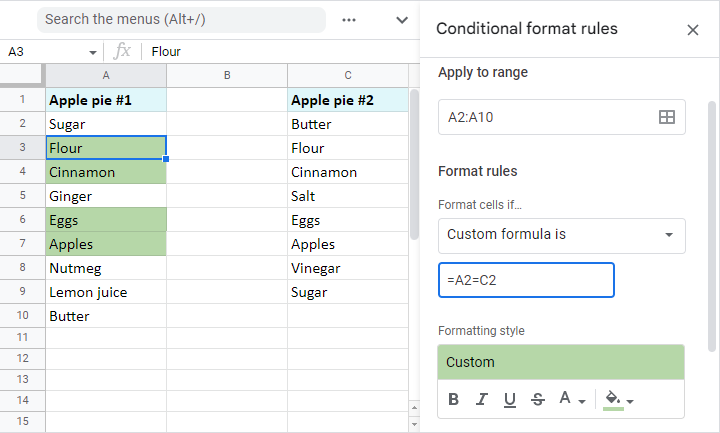Tip. If your columns change in size constantly and you want the rule to consider all new entries, apply it to the entire column (A2:A, assuming the data to compare starts from A2) and modify the formula like this:

`=AND(A2=C2,ISBLANK(A2)=FALSE)`

This will process entire columns and ignore empty cells.

Note. To compare data from two different sheets, you'll have to make other adjustments to the formula. You see, conditional formatting in Google Sheets doesn't support cross-sheet references. However, you can access other sheets indirectly:

`=A2=INDIRECT("Sheet2!C2:C")`

In this case, please make sure to specify the range to apply the rule to – A2:A10.

### Compare two Google sheets and columns for differences

To highlight records that don't match cells on the same row in another column, the drill is the same as above. You select the range and create a conditional formatting rule. However, the formula here differs:

`=A2<>C2`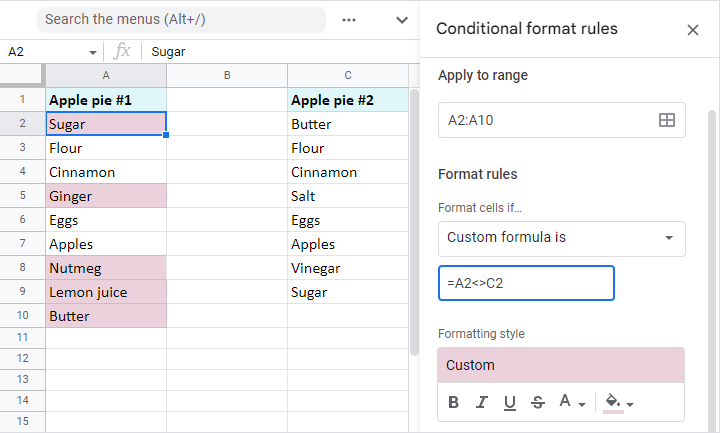Again, modify the formula to make the rule dynamic (have it consider all newly added values in these columns):

`=AND(A2=C2,ISBLANK(A2)=FALSE)`

And use the indirect reference to another sheet if the column to compare with is there:

`=A2<>INDIRECT("Sheet1!C2:C")`

Note. Don't forget to specify the range to apply the rule to – A2:A10.

### Compare two lists and highlight records in both of them

Of course, it's more likely the same records in your columns will be scattered. The value in A2 in one column will not necessarily be on the second row of another column. In fact, it may appear much later. Clearly, this requires another method of searching for the items.

#### Example 1. Compare two columns in Google Sheets and highlight differences (uniques)

To highlight unique values in each list, you need to create two conditional formatting rules for each column.

Color column A: `=COUNTIF(\$C\$2:\$C\$9,\$A2)=0`
Color column C: `=COUNTIF(\$A\$2:\$A\$10,\$C2)=0`

Here are the uniques I've got: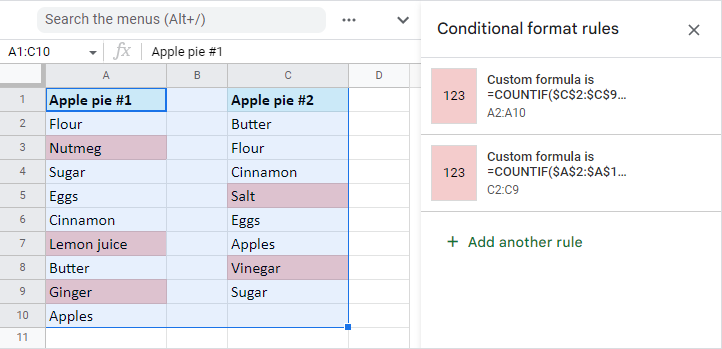#### Example 2. Find and highlight duplicates in two columns in Google Sheets

You can colour common values after slight modifications in both formulas from the previous example. Just make the formula count everything greater than zero.

Color dupes between columns in A only: `=COUNTIF(\$C\$2:\$C\$9,\$A2)>0`
Color dupes between columns in C only: `=COUNTIF(\$A\$2:\$A\$10,\$C2)>0`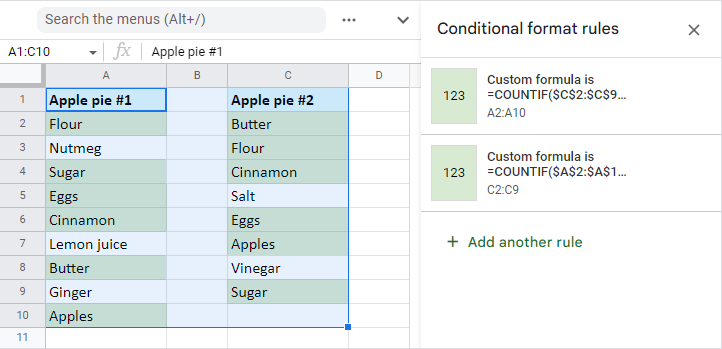### Quick way to match columns and highlight records

Conditional formatting can be tricky sometimes: you may accidentally create a few rules over the same range or apply colors manually over cells with rules. Also, you have to keep an eye on all ranges: the ones you highlight via rules and those you use in rules themselves. All of these may confuse you a lot if you're not prepared and not sure where to look for the problem.

Luckily, our Compare columns or sheets is intuitive enough to help you match two columns within one table, two different tables on one sheet, or even two separate sheets, and highlight those uniques or dupes that may sneak into your data.

Here's how I colored uniques between two tables based on Fruit and MSRP columns using the tool:Tip. Have you seen the video tutorial for the Compare columns or sheets add-on? Check it out.